# Meta-analysis of binary data with baggr: a case study

#### 2020-02-27

This vignette is written for baggr users who want to analyse binary data. For more general introduction to meta-analysis concepts and baggr workflow, please read vignette("baggr").

Here, we will show how to:

• Run meta-analysis of summary odd ratio (OR) or risk ratio (RR) data.
• Prepare standard outputs for the above.
• Convert individual-level data to summary-level and correct for rare events.
• Run meta-analysis directly on individual-level data.
• Model impact of covariates.

## Other questions you should consider

There are certain aspects of modelling binary data that are not yet covered by baggr, but may be important for your data:

# Basic meta-analysis of binary data

Typically, a meta-analysis of binary data is done on summary statistics such as $$\log(OR)$$ or $$\log(RR)$$. The reason for this is two-fold: 1) they are the statistics most commonly reported by studies and 2) they are approximately normally distributed. The second assumption needs to be treated carefully, as we will show later.

For the first example we will use a simple summary data based on (part of) Table 6 in Yusuf et al. (1985), a famous study of impact of beta blockers on occurrence of strokes and mortality.

df_yusuf <- read.table(text="
trial  a n1i  c n2i
Balcon 14  56 15  58
Clausen 18  66 19  64
Multicentre 15 100 12  95
Barber 10  52 12  47
Norris 21 226 24 228
Kahler  3  38  6  31
Ledwich  2  20  3  20
", header=TRUE)

In a typical notation, a (c) are numbers of events in treatment (control) groups, while n1 (n2) are total patients in treatment (control) group. We can also calculate $$b = n_1 - a$$ and $$d = n_2 - c$$, i.e. numbers of “non-events” in treatment and control groups respectively.

In our examples we will use OR metric; $$\log(OR)$$ and its SE can easily be calculated from the values (if you’re not familiar with OR, see here):

df <- df_yusuf
df$b <- df$n1i-df$a df$d <- df$n2i-df$c
df$tau <- log((df$a*df$d)/(df$b*df$c)) df$se <- sqrt(1/df$a + 1/df$b + 1/df$c + 1/df$d)

df
#>         trial  a n1i  c n2i   b   d         tau        se
#> 1      Balcon 14  56 15  58  42  43 -0.04546237 0.4303029
#> 2     Clausen 18  66 19  64  48  45 -0.11860574 0.3888993
#> 3 Multicentre 15 100 12  95  85  83  0.19933290 0.4169087
#> 4      Barber 10  52 12  47  42  35 -0.36464311 0.4855042
#> 5      Norris 21 226 24 228 205 204 -0.13842138 0.3147471
#> 6      Kahler  3  38  6  31  35  25 -1.02961942 0.7540368
#> 7     Ledwich  2  20  3  20  18  17 -0.46262352 0.9735052

We use tau and se notation for our effect, same as we would for analysing continuous data with baggr(). In fact, the model we use for logOR is the same default “Rubin” model (Rubin (1974)) with partial pooling. Once $$\log(OR)$$ and is SE have been calculated, there are no differences between this process and analysis continuous quantities in baggr.

bg_model_agg <- baggr(df,
effect = "logarithm of odds ratio",
group = "trial")

The two arguments we customised, effect and group do not impact results, but make outputs nicer, as we will see in the next section.

As with continuous quantities, the prior is chosen automatically. However, it is important to review the automatic prior choice when analysing logged quantities, because the priors may be needlessly diffuse (in other words, with the command above baggr does not “know” that data are logged).

## Using risk ratios instead of odds ratios

We summarised our data as log(OR). Alternatively, we could work with log(RR), which is also normally distributed and can be easily calculated from contingency tables (i.e. a, b, c, d columns). All of the analysis would be analogous in that case – with exception of interpretation of treatment effect, which will then be on risk ratios.

Quick reminder on how risk and odd ratios compare. If an event is rare (rule of thumb: up to 10%), OR and RR will be similar. For really rare events there is no difference. The higher the event rate, the more discrepancy, e.g.

a <- 9; b <- 1; c <- 99; d <- 1
cat("Risk ratio is", (a/(a+b))/(c/(c+d)), "\n" )
#> Risk ratio is 0.9090909
cat("Odds ratio is", a*d/(b*c), "\n")
#> Odds ratio is 0.09090909
a <- 10; b <- 20; c <- 100; d <- 100
cat("Risk ratio is", (a/(a+b))/(c/(c+d)), "\n" )
#> Risk ratio is 0.6666667
cat("Odds ratio is", a*d/(b*c), "\n")
#> Odds ratio is 0.5

# Visualising and criticising model results

All of the outputs are explained in more detail in the “main” package vignette, vignette("baggr"). Here, I simply illustrate their behaviour.

bg_model_agg
#> Model type: Rubin model with aggregate data
#> Pooling of effects: partial
#>
#> Aggregate treatment effect (on logarithm of odds ratio):
#> Hypermean (tau) =  -0.16 with 95% interval -0.62 to 0.26
#> Hyper-SD (sigma_tau) = 0.248 with 95% interval 0.011 to 0.835
#> Total pooling (1 - I^2) = 0.83 with 95% interval 0.33 to 1.00
#>
#> Treatment effects on logarithm of odds ratio:
#>               mean   sd pooling
#> Balcon      -0.131 0.26    0.76
#> Clausen     -0.142 0.24    0.74
#> Multicentre -0.065 0.27    0.75
#> Barber      -0.198 0.28    0.79
#> Norris      -0.146 0.22    0.68
#> Kahler      -0.263 0.35    0.88
#> Ledwich     -0.177 0.35    0.92

Default plot (plot(bg_model_agg)) will show pooled group results. If you prefer a typical forest plot style, you can use forest_plot, which can also plot comparison with source data (via print argument):

forest_plot(bg_model_agg, show = "both", print = "inputs")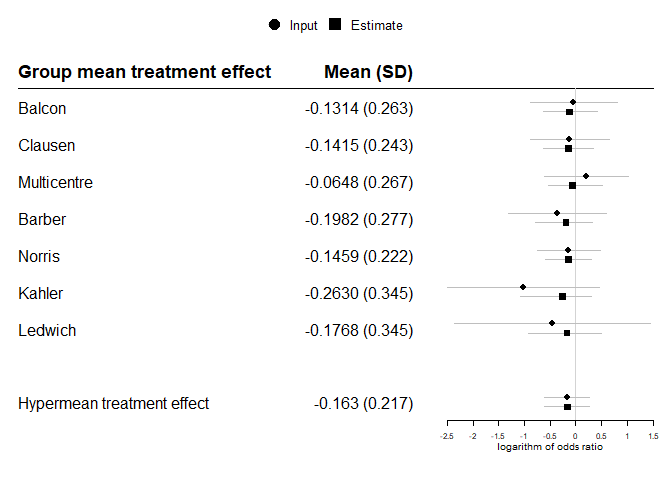Hypothetical treatment effect in a new trial is obtained through effect_plot.

effect_plot(bg_model_agg)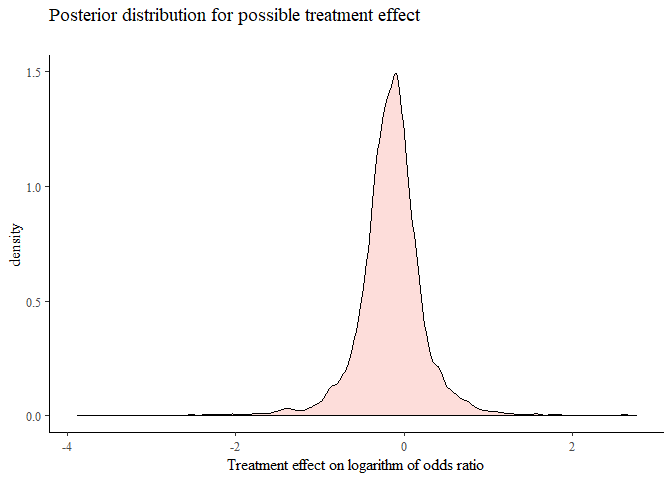We can transform printed and plotted outputs to show exponents (or other transforms); for example:

gridExtra::grid.arrange(
plot(bg_model_agg, transform = exp) + xlab("Effect on OR"),
effect_plot(bg_model_agg, transform = exp) + xlim(0, 3) + xlab("Effect on OR"),
ncol = 2)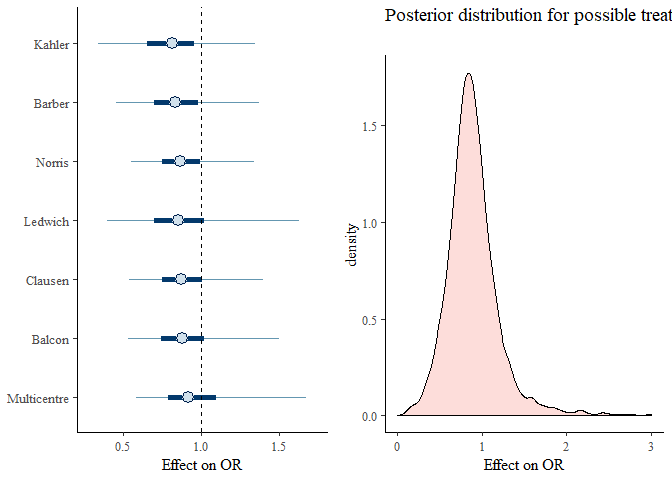## Model comparison

We can compare with no pooling and full pooling models using baggr_compare

# Instead of writing...
# bg1 <- baggr(df, pooling = "none")
# bg2 <- baggr(df, pooling = "partial")
# bg3 <- baggr(df, pooling = "full")

# ...we use this one-liner
bg_c <- baggr_compare(df, effect = "logarithm of odds ratio")
plot(bg_c)
#> []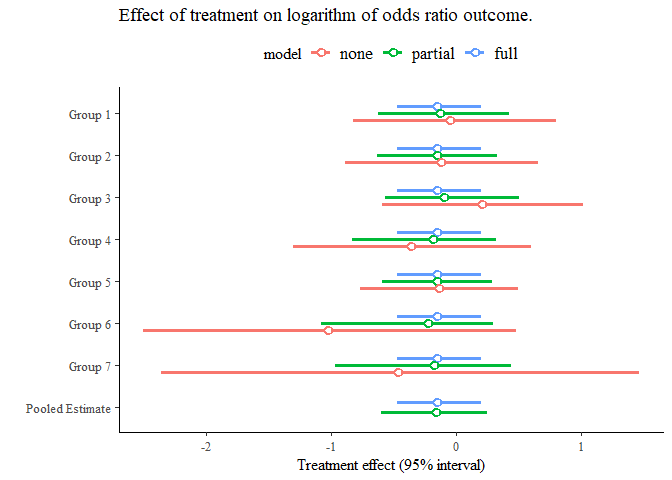#>
#> attr(,"class")
#>  "plot_list"

We can also examine the compared models directly, by accessing them via $models. This is useful e.g. for effect_plot: effect_plot( "Partial pooling, default prior" = bg_c$models$partial, "Full pooling, default prior" = bg_c$models$full) + theme(legend.position = "bottom")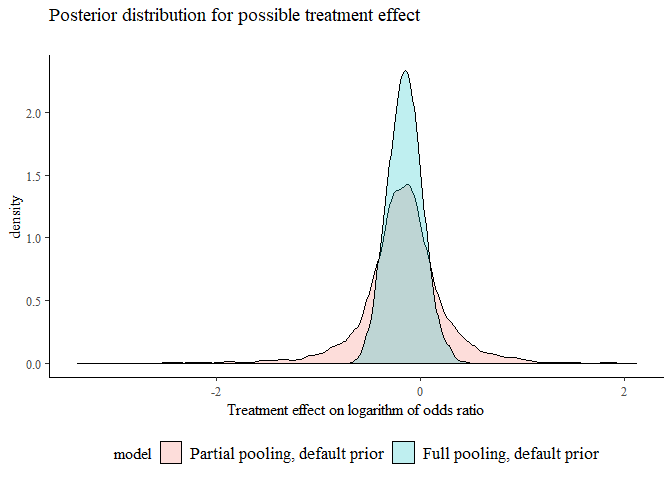You can use leave-one-out cross-validation to compare the models quantitatively. See documentation of ?loocv for more details of calculation. a <- loocv(df, pooling = "partial") b <- loocv(df, pooling = "full") #a; b; #you can print out individual loocv() calculations loo_compare(a,b) #...but typically we compare them to each other # Model for individual-level binary data Let’s assume that you have access to underlying individual-level data. In our example above, we do not, but we can create a data.frame with individual level data as we know the contingency table: df_ind <- data.frame() for(i in 1:nrow(df_yusuf)) { df_ind <- rbind(df_ind, data.frame(group = df_yusuf$trial[i],
treatment = c(rep(1, df_yusuf$n1i[i]), rep(0, df_yusuf$n2i[i])),
outcome = c(rep(1, df_yusuf$a[i]), rep(0, df_yusuf$n1i[i] - df_yusuf$a[i]), rep(1, df_yusuf$c[i]), rep(0, df_yusuf$n2i[i] - df_yusuf$c[i]))))
}
#>    group treatment outcome
#> 1 Balcon         1       1
#> 2 Balcon         1       1
#> 3 Balcon         1       1
#> 4 Balcon         1       1
#> 5 Balcon         1       1
#> 6 Balcon         1       1

We can now use a logistic regression model

bg_model_ind <- baggr(df_ind, model = "logit", effect = "logarithm of odds ratio")

Note: as in other cases, baggr() will detect model appropriately (in this case by noting that outcome has only 0’s and 1’s) and notify the user, so in everyday use, it is not necessary to set model = "logit" .

If we denote binary outcome as $$y$$, treatment as $$z$$ (both indexed over individual units by $$i$$), under partial pooling the logistic regression model assumes that

$y_i \sim \text{Bernoulli}(\text{logit}^{-1}[\mu_{\text{group}(i)} + \tau_{\text{group}(i)}z_i])$

where $$\mu_k$$’s and $$\tau_k$$’s are parameters to estimate. The former is a nuisance parameter, defining a group-specific mean probability of event, without treatment. The latter are group-specific effects.

Under this formulation, odds ratio of event between treatment and non-treatment are given by $$\tau_k$$. This means, the modelling assumption is the same as when working with summarised data. Moreover, baggr’s default prior for $$\tau_k$$ is set in the same way for summary and individual-level data (you can see it as bg_model_ind$formatted_prior). One minor difference is that parameter $$\mu$$ is estimated, but unless dealing with more extreme values of $$\mu$$, i.e. with rare or common events, this should not impact the modelled result. Therefore, the result we get from the two models will be very close (albeit with some numerical variation due to short run time): baggr_compare(bg_model_agg, bg_model_ind) #> Mean treatment effects: #> 2.5% mean 97.5% median sd #> Model 1 -0.620931 -0.163213 0.263314 -0.156234 0.217500 #> Model 2 -0.596394 -0.177299 0.221972 -0.169348 0.211027 #> #> SD for treatment effects: #> 2.5% mean 97.5% median sd #> Model 1 0.01122390 0.247723 0.834529 0.186882 0.225403 #> Model 2 0.00734586 0.246008 0.822508 0.186327 0.218467 #> #> Transform: NULL There will be difference in speed – in our example logistic model has to work with 1101 rows of data, while the summary level model only has 7 datapoints. Therefore you are better off summarising your data and using the default model, which I will show next. ## When to use summary and when to use individual-level data To sum up: • If you have no covariates or a particular type of priors (e.g. on baseline risk), you are better off using a summary-level model. I will show how you can summarise your data in the next section. • If your data includes covariates or events are rare, you should consider the logistic model. I will show why below. Note: as shown above, you can create data for logistic model from a, b/n1, c, d/n2 columns. You cannot do it from OR only, as the denominator is missing. # Summarising individual-level data In the previous example we analysed individual-level data. It is useful to summarise it, either to speed up modelling (see above) or to better understand the inputs. The generic function for doing this in baggr is prepare_ma. It can be used with both continuous and binary data. In our case we can summarise df_ind to obtain either odds ratios or risk ratios: prepare_ma(df_ind, effect = "logOR") #> group a n1 c n2 b d tau se #> 1 Balcon 14 56 15 58 42 43 -0.04546237 0.4303029 #> 2 Barber 10 52 12 47 42 35 -0.36464311 0.4855042 #> 3 Clausen 18 66 19 64 48 45 -0.11860574 0.3888993 #> 4 Kahler 3 38 6 31 35 25 -1.02961942 0.7540368 #> 5 Ledwich 2 20 3 20 18 17 -0.46262352 0.9735052 #> 6 Multicentre 15 100 12 95 85 83 0.19933290 0.4169087 #> 7 Norris 21 226 24 228 205 204 -0.13842138 0.3147471 prepare_ma(df_ind, effect = "logRR") #> group a n1 c n2 b d tau se #> 1 Balcon 14 56 15 58 42 43 -0.03390155 0.3209310 #> 2 Barber 10 52 12 47 42 35 -0.28341767 0.3779232 #> 3 Clausen 18 66 19 64 48 45 -0.08483888 0.2782276 #> 4 Kahler 3 38 6 31 35 25 -0.89674614 0.6643991 #> 5 Ledwich 2 20 3 20 18 17 -0.40546511 0.8563488 #> 6 Multicentre 15 100 12 95 85 83 0.17185026 0.3598245 #> 7 Norris 21 226 24 228 205 204 -0.12472076 0.2836811 In both cases the effect (OR or RR) and its SE were renamed to tau and se, so that the resulting data frame can be used directly as input to baggr(). # Rare events vs summarised data Consider data on four fictional studies: df_rare <- data.frame(group = paste("Study", LETTERS[1:4]), a = c(0, 2, 1, 3), c = c(2, 2, 3, 3), n1i = c(120, 300, 110, 250), n2i = c(120, 300, 110, 250)) In Study 1 you can see that no events occurred in a column. Assume, that we have individual-level data for these studies (if not, we can generate it as we did in the example above): df_rare_ind <- data.frame() for(i in 1:nrow(df_rare)) { df_rare_ind <- rbind(df_rare_ind, data.frame(group = df_rare$group[i],
treatment = c(rep(1, df_rare$n1i[i]), rep(0, df_rare$n2i[i])),
outcome = c(rep(1, df_rare$a[i]), rep(0, df_rare$n1i[i] - df_rare$a[i]), rep(1, df_rare$c[i]), rep(0, df_rare$n2i[i] - df_rare$c[i]))))
}

Function prepare_ma() can be used to summarise the rates and calculate log OR/RR. It can also be used to apply corrections to event rates:

df_rare_logor <- prepare_ma(df_rare_ind, effect = "logOR")
df_rare_logor
#>     group    a     n1    c     n2      b      d       tau        se
#> 1 Study A 0.25 120.25 2.25 120.25 120.25 118.25 -2.213996 2.1121593
#> 2 Study B 2.00 300.00 2.00 300.00 298.00 298.00  0.000000 1.0033501
#> 3 Study C 1.00 110.00 3.00 110.00 109.00 107.00 -1.117131 1.1626923
#> 4 Study D 3.00 250.00 3.00 250.00 247.00 247.00  0.000000 0.8214401

Note that the output of prepare_ma now differs from the original df_rare for “Study A”: a (default) value of 0.25 was added, because there were no events in treatment arm. That means $$\log(OR)=\log(0)=-\infty$$. It is typical to correct for rare events when analysing summary level data. A great overview of the subject and how different meta-analysis methods perform is provided by Bradburn et al. (2007). You can modify the amount of correction by setting rare_event_correction argument.

pma01 <- prepare_ma(df_rare_ind, effect = "logOR",
rare_event_correction = 0.1)
pma1 <- prepare_ma(df_rare_ind, effect = "logOR",
rare_event_correction = 1)

When rare events occur, you can try to understand the impact of correction by comparing models with different rare_event_correction values:

bg_correction01 <- baggr(pma01, effect = "logOR")
bg_correction025 <- baggr(df_rare_logor, effect = "logOR")
bg_correction1 <- baggr(pma1, effect = "logOR")
bg_rare_ind <- baggr(df_rare_ind, effect = "logOR")
bgc <- baggr_compare(
"Correct by .10" = bg_correction01,
"Correct by .25" = bg_correction025,
"Correct by 1.0" = bg_correction1,
"Individual data" = bg_rare_ind
)
bgc
#> Mean treatment effects:
#>                     2.5%      mean   97.5%    median       sd
#> Correct by .10  -2.80528 -0.460443 1.78505 -0.446145 1.092570
#> Correct by .25  -2.30077 -0.493740 1.29194 -0.457582 0.866460
#> Correct by 1.0  -2.29699 -0.534690 0.97479 -0.491637 0.818131
#> Individual data -4.37018 -0.984133 1.39341 -0.803722 1.346270
#>
#> SD for treatment effects:
#>                      2.5%     mean   97.5%   median       sd
#> Correct by .10  0.0358399 1.448000 5.76854 0.930287 1.524060
#> Correct by .25  0.0428817 1.181230 4.49493 0.803533 1.270460
#> Correct by 1.0  0.0202896 0.971861 3.66782 0.693716 0.955043
#> Individual data 0.0650471 1.927440 7.44016 1.286640 1.960770
#>
#> Transform: NULL
plot(bgc)
#> []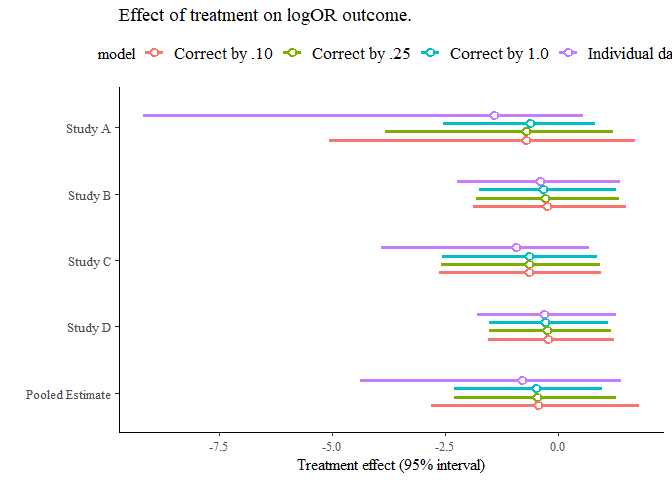#>
#> attr(,"class")
#>  "plot_list"

However, the issue with modelling rare events is not limited to zero-events. As mentioned, $$\log(OR)$$ is approximately Gaussian. The quality of the approximation will depend on probabilities in all cells of the contingency table (which we estimate through a, b, c, d). Therefore, treating $$\log(OR)$$ as Gaussian might lead to different results in the individual-level vs summary-level models if events are rare. With the low counts in our example it will definitely be the case.

Let us generate new data without 0’s, so that you can see how 0’s are not the issue:

df_rare <- data.frame(group = paste("Study", LETTERS[1:4]),
a = c(1, 2, 1, 3), c = c(2, 2, 3, 3),
n1i = c(120, 300, 110, 250),
n2i = c(120, 300, 110, 250))

df_rare_ind <- data.frame()

for(i in 1:nrow(df_rare)) {
df_rare_ind <- rbind(df_rare_ind, data.frame(group = df_rare$group[i], treatment = c(rep(1, df_rare$n1i[i]), rep(0, df_rare$n2i[i])), outcome = c(rep(1, df_rare$a[i]), rep(0, df_rare$n1i[i] - df_rare$a[i]),
rep(1, df_rare$c[i]), rep(0, df_rare$n2i[i] - df_rare$c[i])))) } df_rare_logor <- prepare_ma(df_rare_ind, effect = "logOR") bg_rare_agg <- baggr(df_rare_logor, effect = "logOR") bg_rare_ind <- baggr(df_rare_ind, effect = "logOR") Let’s compare again, both on group level but also in terms of hypothetical treatment effect: bgc <- baggr_compare( "Summary-level (Rubin model on logOR)" = bg_rare_agg, "Individual-level (logistic model)" = bg_rare_ind ) bgc #> Mean treatment effects: #> 2.5% mean 97.5% median #> Summary-level (Rubin model on logOR) -1.80662 -0.325010 1.38703 -0.339441 #> Individual-level (logistic model) -2.49763 -0.477669 1.39086 -0.444139 #> sd #> Summary-level (Rubin model on logOR) 0.764156 #> Individual-level (logistic model) 0.907515 #> #> SD for treatment effects: #> 2.5% mean 97.5% median #> Summary-level (Rubin model on logOR) 0.0272445 0.934829 3.57101 0.653308 #> Individual-level (logistic model) 0.0305191 1.099900 3.95634 0.777828 #> sd #> Summary-level (Rubin model on logOR) 0.90796 #> Individual-level (logistic model) 1.02539 #> #> Transform: NULL plot(bgc) #> []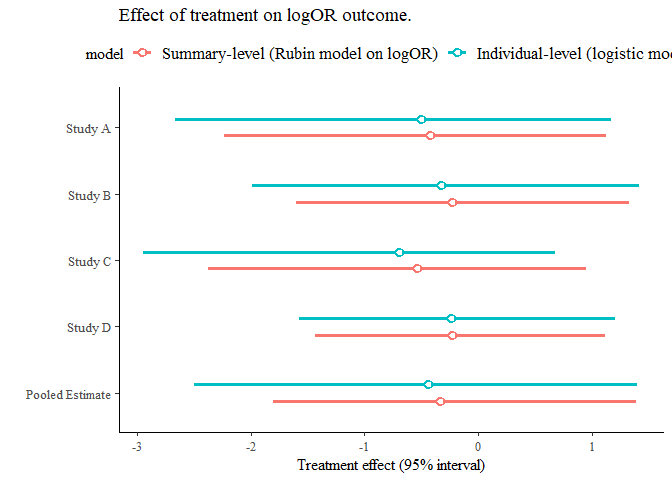#> #> attr(,"class") #>  "plot_list" The results are still a bit different. # Accounting for covariates: meta-regression and mixed models This section provides only a short example. To learn more about meta-regression see e.g. Baker et al. (2009) Two types of covariates may be present in your data: • Covariates that change according to group unit. In that case, the model accounting for the group covariates is a meta-regression model. It can be modelled on summary-level data. • Covariates that change according to individual unit. Then, the model can be called a mixed model. It has to be fitted to individual-level data. Note that the first case can also be accounted for by using a mixed model. In both cases we only need to name one extra argument to baggr: covariates=, followed by a vector of column names in input data. You should remember that your treatment effect estimate will vary a lot not only with choice of covariates, but also the contrasts that you use. For example: #let's use the data.frame we created from Yusuf et al earlier df$study_grouping      <- c(1,1,1,0,0,0,0)
df\$different_contrasts <- c(1,1,1,0,0,0,0) - .5
bg_cov1 <- baggr(df, covariates = c("study_grouping"), effect = "logarithm of odds ratio")
bg_cov2 <- baggr(df, covariates = c("different_contrasts"), effect = "logarithm of odds ratio")
baggr_compare("No covariate" = bg_model_agg,
"With covariates, 0-1 coding" = bg_cov1,
"With covariates, +-1/2 coding" = bg_cov2)
#> Mean treatment effects:
#>                                    2.5%      mean    97.5%    median       sd
#> No covariate                  -0.620931 -0.163213 0.263314 -0.156234 0.217500
#> With covariates, 0-1 coding   -1.052960 -0.351897 0.310404 -0.346460 0.335913
#> With covariates, +-1/2 coding -0.653897 -0.176452 0.260016 -0.172070 0.227764
#>
#> SD for treatment effects:
#>                                    2.5%     mean    97.5%   median       sd
#> No covariate                  0.0112239 0.247723 0.834529 0.186882 0.225403
#> With covariates, 0-1 coding   0.0125879 0.294858 1.028340 0.220150 0.273762
#> With covariates, +-1/2 coding 0.0115398 0.284586 0.965491 0.215459 0.257894
#>
#> Transform: NULL

To access the covariate estimates, see fixed_effects() function. They are also printed out by default:

bg_cov1
#> Model type: Rubin model with aggregate data
#> Pooling of effects: partial
#>
#> Aggregate treatment effect (on logarithm of odds ratio):
#> Hypermean (tau) =  -0.35 with 95% interval -1.05 to 0.31
#> Hyper-SD (sigma_tau) = 0.295 with 95% interval 0.013 to 1.028
#> Total pooling (1 - I^2) = 0.79 with 95% interval 0.24 to 1.00
#>
#> Treatment effects on logarithm of odds ratio:
#>          mean   sd pooling
#> Group 1 -0.36 0.43    0.72
#> Group 2 -0.39 0.42    0.69
#> Group 3 -0.29 0.42    0.71
#> Group 4 -0.34 0.32    0.75
#> Group 5 -0.26 0.27    0.63
#> Group 6 -0.44 0.41    0.85
#> Group 7 -0.35 0.43    0.89
#>
#> Covariate (fixed) effects on logarithm of odds ratio:
#>      [,1]
#> mean 0.36
#> sd   0.45